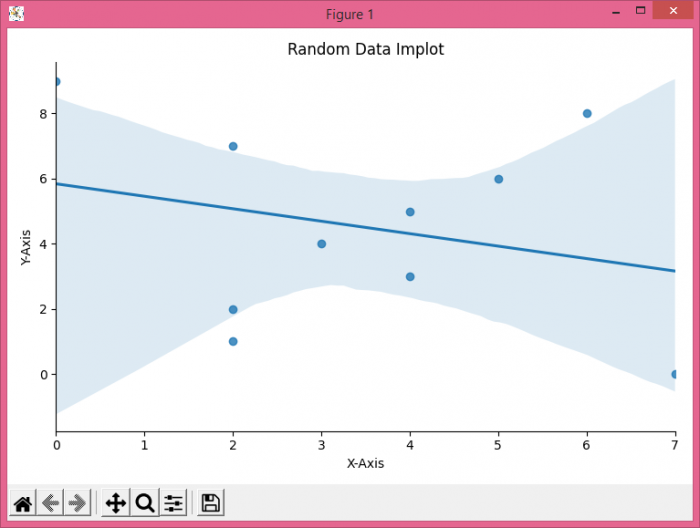# How to add a title on Seaborn lmplot?

To add a title on Seaborn Implot, we can take the following steps−

• Set the figure size and adjust the padding between and around the subplots.
• Make a Pandas dataframe with two columns, X-Axis and Y-Axis
• Use implot() method.
• Get the current axis using gca() method.
• To display the figure, use show() method.

## Example

import pandas
import matplotlib.pylab as plt
import seaborn as sns
import numpy as np

plt.rcParams["figure.figsize"] = [7.50, 3.50]
plt.rcParams["figure.autolayout"] = True

df = pandas.DataFrame({"X-Axis": [np.random.randint(10) for i in range(10)], "Y-Axis": [i for i in range(10)]})

bar_plot = sns.lmplot(x='X-Axis', y='Y-Axis', data=df, height=3.5)

ax = plt.gca()

ax.set_title("Random Data Implot")

plt.show()

## Output